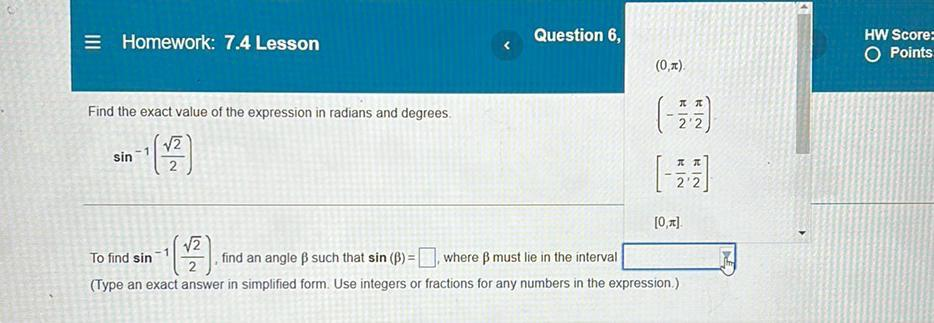Question:

# = Homework: 7.4 Lesson Question 6, HW Score: O Points (0,2) Find the exact value of the expression in radians and degrees V2 sin= Homework: 7.4 Lesson Question 6, HW Score: O Points (0,2) Find the exact value of the expression in radians and degrees V2 sin (7) () KIN kle  V2 To find sin find an angle B such that sin (8)= where ß must lie in the interval (Type an exact answer in simplified form. Use integers or fractions for any numbers in the expression) =